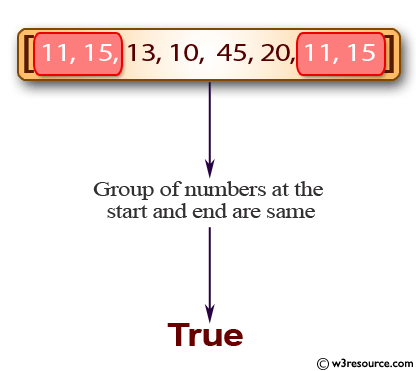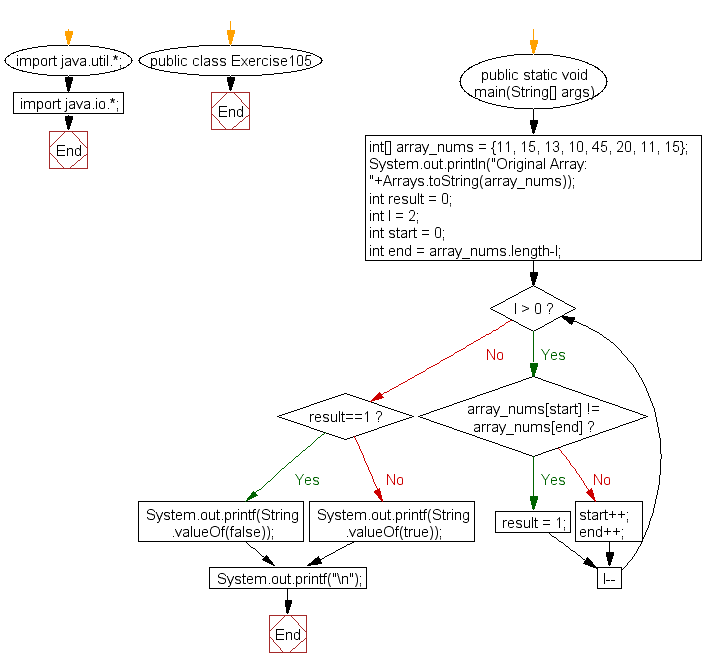﻿ Java: Verify the start and end of an array are the same# Java Exercises: Check if a group of numbers at the start and end of a given array are same

## Java Basic: Exercise-105 with Solution

Write a Java program to check if a group of numbers (l) at the start and end of a given array are same.

Pictorial Presentation:Sample Solution:

Java Code:

``````import java.util.*;
import java.io.*;
public class Exercise105 {
public static void main(String[] args)
{
int[] array_nums = {11, 15, 13, 10, 45, 20, 11, 15};
System.out.println("Original Array: "+Arrays.toString(array_nums));

int result = 0;
int l = 2;
int start = 0;
int end = array_nums.length-l;
for(; l > 0; l--)
{
if(array_nums[start] != array_nums[end])
result = 1;
else
{
start++;
end++;
}
}

if (result==1)
{
System.out.printf(String.valueOf(false));
}
else
{
System.out.printf(String.valueOf(true));
}
System.out.printf("\n");
}
}
```
```

Sample Output:

```Original Array: [11, 15, 13, 10, 45, 20, 11, 15]
true
```

Flowchart:Java Code Editor:

What is the difficulty level of this exercise?

Test your Programming skills with w3resource's quiz.

﻿

## Java: Tips of the Day

countOccurrences

Counts the occurrences of a value in an array.

Use Arrays.stream().filter().count() to count total number of values that equals the specified value.

```public static long countOccurrences(int[] numbers, int value) {
return Arrays.stream(numbers)
.filter(number -> number == value)
.count();
}
```

Ref: https://bit.ly/3kCAgLb